I used the following to put this document on the wiki:

``````library(wwiki)
pushWiki("CA_homicide.rmd", wiki = "bio_708", user = "Bb", page = "homicide_example")
``````

## Read data in wide format and play with it

Data are originally from here (it might be possible to scrape this data set using the `XML` package …).

``````dat <- read.csv("CA_homicide.csv", check.names = FALSE)
``````

We use `check.names=FALSE` to stop R from trying to sanitize the column names: this is reasonable if we plan to convert to long form and want to preserve the values (years, in this case).

These data are in wide format:

``````head(dat)
``````
``````##                       Place 2007 2008 2009 2010 2011
## 1                    Canada 1.80 1.83 1.81 1.62 1.73
## 2 Newfoundland and Labrador 0.59 0.99 0.20 0.78 0.78
## 3      Prince Edward Island 0.00 1.43 0.00 0.00 0.69
## 4               Nova Scotia 1.39 1.28 1.60 2.22 2.33
## 5             New Brunswick 1.07 0.40 1.60 1.20 1.06
## 6                    Quebec 1.17 1.19 1.12 1.06 1.32
``````

What if we want combine other information?

``````rdat <- data.frame(Place = dat\$Place, Region = c("all", rep("Atlantic", 4),
rep("East", 2), rep("West", 4), rep("North", 3)))
dat2 <- merge(dat, rdat)
``````
``````##                       Place 2007 2008 2009 2010 2011   Region
## 1                   Alberta 2.51 3.06 2.59 2.07 2.88     West
## 2          British Columbia 2.04 2.67 2.65 1.83 1.90     West
## 3                    Canada 1.80 1.83 1.81 1.62 1.73      all
## 4                  Manitoba 5.11 4.48 4.68 3.65 4.24     West
## 5             New Brunswick 1.07 0.40 1.60 1.20 1.06 Atlantic
## 6 Newfoundland and Labrador 0.59 0.99 0.20 0.78 0.78 Atlantic
``````

Since we have the rows in the same order, we could just say `dat\$Region <- rdat\$Region`, but this is much safer – what if we got the row order mixed up by accident?

It's fairly easy to get summary statistics by dropping the first row (so that we have a data frame that is all numeric) and computing means of rows and columns:

``````dmat <- dat[, -1]
rownames(dmat) <- dat[, 1]
rowMeans(dmat)  ## means by place
``````
``````##                    Canada Newfoundland and Labrador
##                     1.758                     0.668
##      Prince Edward Island               Nova Scotia
##                     0.424                     1.764
##             New Brunswick                    Quebec
##                     1.066                     1.172
##                   Ontario                  Manitoba
##                     1.386                     4.432
##                     3.262                     2.622
##          British Columbia                     Yukon
##                     2.218                     4.806
##     Northwest Territories                   Nunavut
##                     5.038                    18.584
``````
``````colMeans(dmat)  ## means by year
``````
``````##  2007  2008  2009  2010  2011
## 3.812 3.588 3.589 3.040 3.543
``````

(Don't forget the `na.rm` argument, unnecessary in this case, that can be provided to most R summary functions to get them to ignore `NA` values.)

We can make a (very ugly) plot fairly simply by using `t()` to transpose the data so that columns, rather than rows, represent places – this is what R's `matplot()` function expects:

``````tdat <- t(dmat)
matplot(tdat)
``````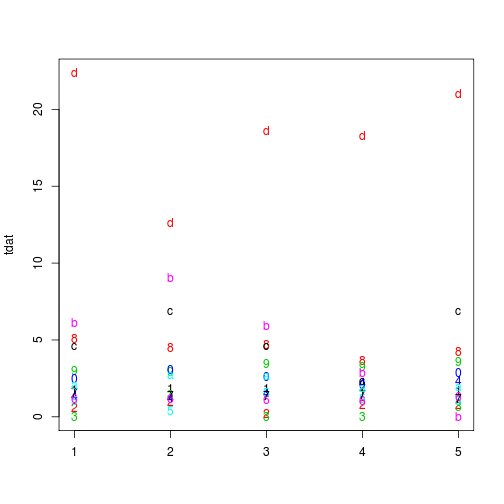We can make it a little bit prettier by

• specifying an `x`-axis variable (the default is just to number the values by their row number)
• using lines instead of points
• provide `x` and `y` axis labels
• using a log scale for the `y` axis
• tweaking graphics parameters with `par` to make the `y`-axis labels horizontal; remove the lines on the top and right of the graph area; and squeeze the axis labels a little bit closer to the plot
``````par(las = 1, bty = "l", mgp = c(2.5, 1, 0))
matplot(2007:2011, tdat, type = "l", xlab = "year", ylab = "Homicide rate per 100,000",
log = "y")
``````
``````## Warning: 4 y values <= 0 omitted from logarithmic plot
``````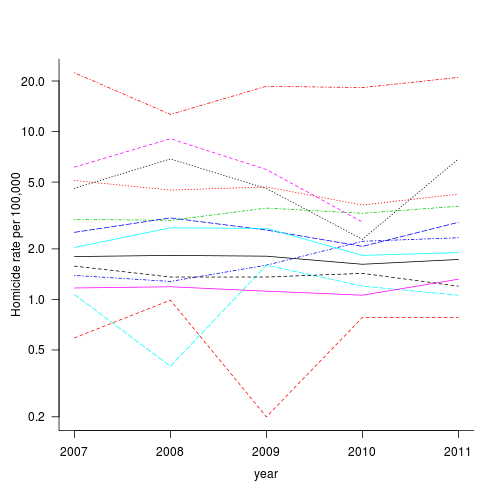(note that we get a warning message about the zero values that have been dropped …)

The `legend` function would help improve the graph too – but we are going to have some trouble distinguishing 14 different lines in the same plot anyway … we will pursue a different approach.

What would the plot look like/mean if we hadn't transposed the data matrix?

## Long format

``````library(reshape)
## reshape data from wide to long ('melt')
mdat <- melt(dat, id.vars = 1, variable_name = "Year")
mdat <- rename(mdat, c(value = "Hom_rate"))
## translate years back to a numeric variable
mdat\$Year <- as.numeric(as.character(mdat\$Year))
``````

We can also `merge` even if we have the data in long format:

``````mdat <- merge(mdat, rdat)
``````
``````##              Place Year Hom_rate Region
## 1          Alberta 2011     2.88   West
## 2          Alberta 2010     2.07   West
## 3          Alberta 2009     2.59   West
## 4          Alberta 2008     3.06   West
## 5          Alberta 2007     2.51   West
## 6 British Columbia 2010     1.83   West
``````
``````mdat <- merge(mdat, popdat)
``````
``````##              Place Year Hom_rate Region Pop_2011
## 1          Alberta 2011     2.88   West  3645257
## 2          Alberta 2010     2.07   West  3645257
## 3          Alberta 2009     2.59   West  3645257
## 4          Alberta 2008     3.06   West  3645257
## 5          Alberta 2007     2.51   West  3645257
## 6 British Columbia 2010     1.83   West  4400057
``````

One more useful technique is reordering factors (representing categorical variables) in a sensible way. Right now the 'places' (provinces, territories, etc.) are ordered alphabetically, R's default.

``````mdat\$Place <- reorder(mdat\$Place, mdat\$Pop_2011)
``````

## Casting

How do we summarize the data if we have it in long format?

Mean by place:

``````place_mean <- cast(mdat, Place ~ ., value = "Hom_rate", fun.aggregate = mean)
names(place_mean) <- "mean_hom"
print(place_mean)
``````
``````##                        Place mean_hom
## 1                    Nunavut   18.584
## 2                      Yukon    4.806
## 3      Northwest Territories    5.038
## 4       Prince Edward Island    0.424
## 5  Newfoundland and Labrador    0.668
## 6              New Brunswick    1.066
## 7                Nova Scotia    1.764
## 9                   Manitoba    4.432
## 10                   Alberta    2.622
## 11          British Columbia    2.218
## 12                    Quebec    1.172
## 13                   Ontario    1.386
``````

By year:

``````cast(mdat, Year ~ ., value = "Hom_rate", fun.aggregate = mean)
``````
``````##   Year (all)
## 1 2007 3.812
## 2 2008 3.588
## 3 2009 3.589
## 4 2010 3.040
## 5 2011 3.543
``````

By region:

``````cast(mdat, Region ~ ., value = "Hom_rate", fun.aggregate = mean)
``````
``````##     Region  (all)
## 1      all 1.7580
## 2 Atlantic 0.9805
## 3     East 1.2790
## 4    North 9.4760
## 5     West 3.1335
``````

I can get multiple summaries in the same data frame: R doesn't have a built in standard error function so I will define one:

``````sem <- function(x) {
sd(x)/sqrt(length(x))
}
cast(mdat, Region ~ ., value = "Hom_rate", fun.aggregate = c(mean, sem))
``````
``````##     Region   mean     sem
## 1      all 1.7580 0.03839
## 2 Atlantic 0.9805 0.15213
## 3     East 1.2790 0.05028
## 4    North 9.4760 1.87790
## 5     West 3.1335 0.21001
``````

We could also cast it back into the original wide format, or into the transposed form, using `cast(mdat,Year~Place)` or `cast(mdat,Place~Year)`. `reshape` can do considerably more complicated things, too.

In the long run it is generally easier to keep your data in long format and cast it to wide as necessary.

`plyr` equivalent:

``````library(plyr)
ddply(mdat, "Region", summarize, mean = mean(Hom_rate), sem = sem(Hom_rate))
``````
``````##     Region   mean     sem
## 1      all 1.7580 0.03839
## 2 Atlantic 0.9805 0.15213
## 3     East 1.2790 0.05028
## 4    North 9.4760 1.87790
## 5     West 3.1335 0.21001
``````
``````dat3 <- merge(merge(place_mean, popdat), rdat)
par(las = 1, bty = "l")
with(dat3, plot(Pop_2011, mean_hom, pch = 16, log = "xy", col = Region))
``````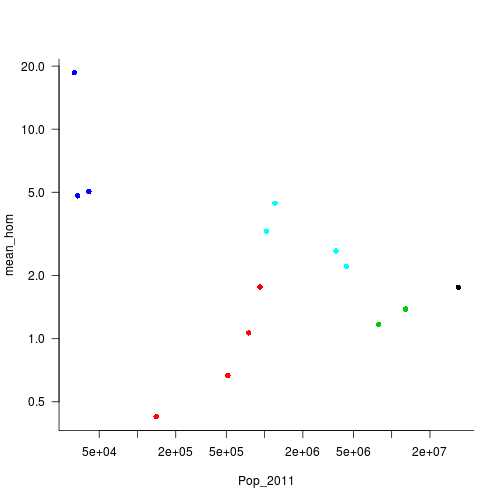### Pictures from long format

One of the other advantages of long format is that it allows us to use some of R's more powerful graphics tools such as the `ggplot2` and `lattice` packages (and it's what most statistics packages expect):

``````library(ggplot2)
theme_set(theme_bw())
p1 <- qplot(Year, Hom_rate, colour = Place, data = mdat, geom = "line") + scale_y_log10() +
labs(y = "Homicides per 100,000 population")
print(p1)
``````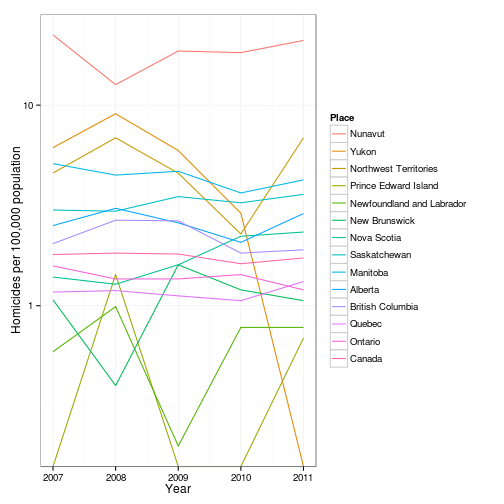Using the directlabels package:

``````library(directlabels)
direct.label(p1) + expand_limits(x = 2004, y = 0.1)
``````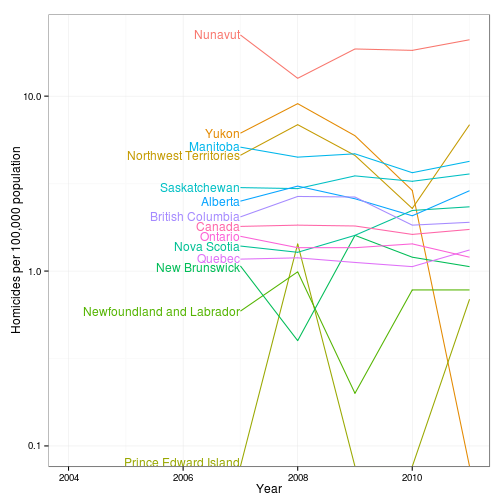Still needs work … should facet by pop size, place, etc.

``````p2 <- ggplot(mdat, aes(Year, Hom_rate, colour = Region, size = log(Pop_2011),
group = Place)) + geom_line(alpha = 0.5) + scale_y_log10() + labs(y = "Homicides per 100,000 population")
print(p2)
``````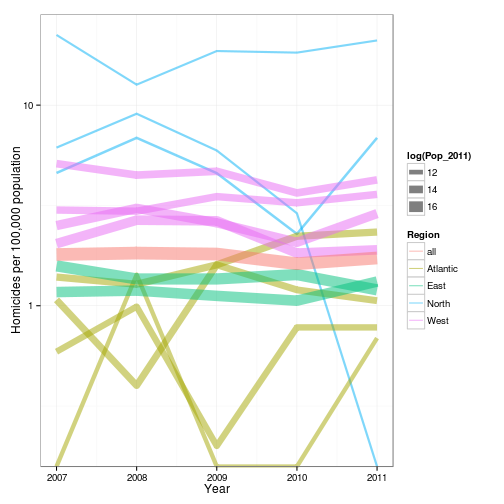(Can't quite get direct labeling to work with this)